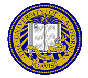# Lecture 13: October 23, 2019

Due: Lab 2, due November 6, 2019; Homework 2, due October 21, 2019

1. Greetings and felicitations!

2. Puzzle of the Day

3. Use of public key cryptosystem
1. Normally used as key interchange system to exchange secret keys (cheap)
2. Then use secret key system (too expensive to use public key cryptosystem for this)

4. RSA
1. Provides both authenticity and confidentiality
2. Go through algorithm:
Idea: C = Me mod n, M = Cd mod n, with ed mod φ(n) = 1
Public key is (e, n); private key is d. Choose n = pq; then φ(n) = (p−1)(q−1).
3. Example: p = 5, q = 7; then n = 35, φ(n) = (5−1)(7−1) = 24. Pick d = 11.
Then ed mod φ(n) = 1, so e = 11
To encipher 2, C = Me mod n = 211 mod 35 = 2048 mod 35 = 18, and M = Cd mod n = 1811 mod 35 = 2.
4. Example: p = 53, q = 61; then n = 3233, φ(n) = (53−1)(61−1) = 3120. Pick d = 791. Then e = 71
To encipher M = RENAISSANCE, use the mapping A = 00, B = 01, …, Z = 25, (space) = 26.
Then: M = RE NA IS SA NC E␢ = 1704 1300 0818 1800 1302 0426
So: C = 170471 mod 3233 = 3106; … = 3106 0100 0931 2691 1984 2927
5. Cryptographic Checksums
1. Function y = h(x): easy to compute y given x; computationally infeasible to compute x given y
2. Variant: given x and y, computationally infeasible to find a second x′ such that y = h(x′)
3. Keyed vs. keyless

6. Digital Signatures
1. Judge can confirm, to the limits of technology, that claimed signer did sign message
2. RSA digital signatures: sign, then encipher, then signMatt Bishop Office: 2209 Watershed Sciences Phone: +1 (530) 752-8060 Email: mabishop@ucdavis.edu
ECS 153, Computer Security
Version of December 1, 2019 at 12:58AM